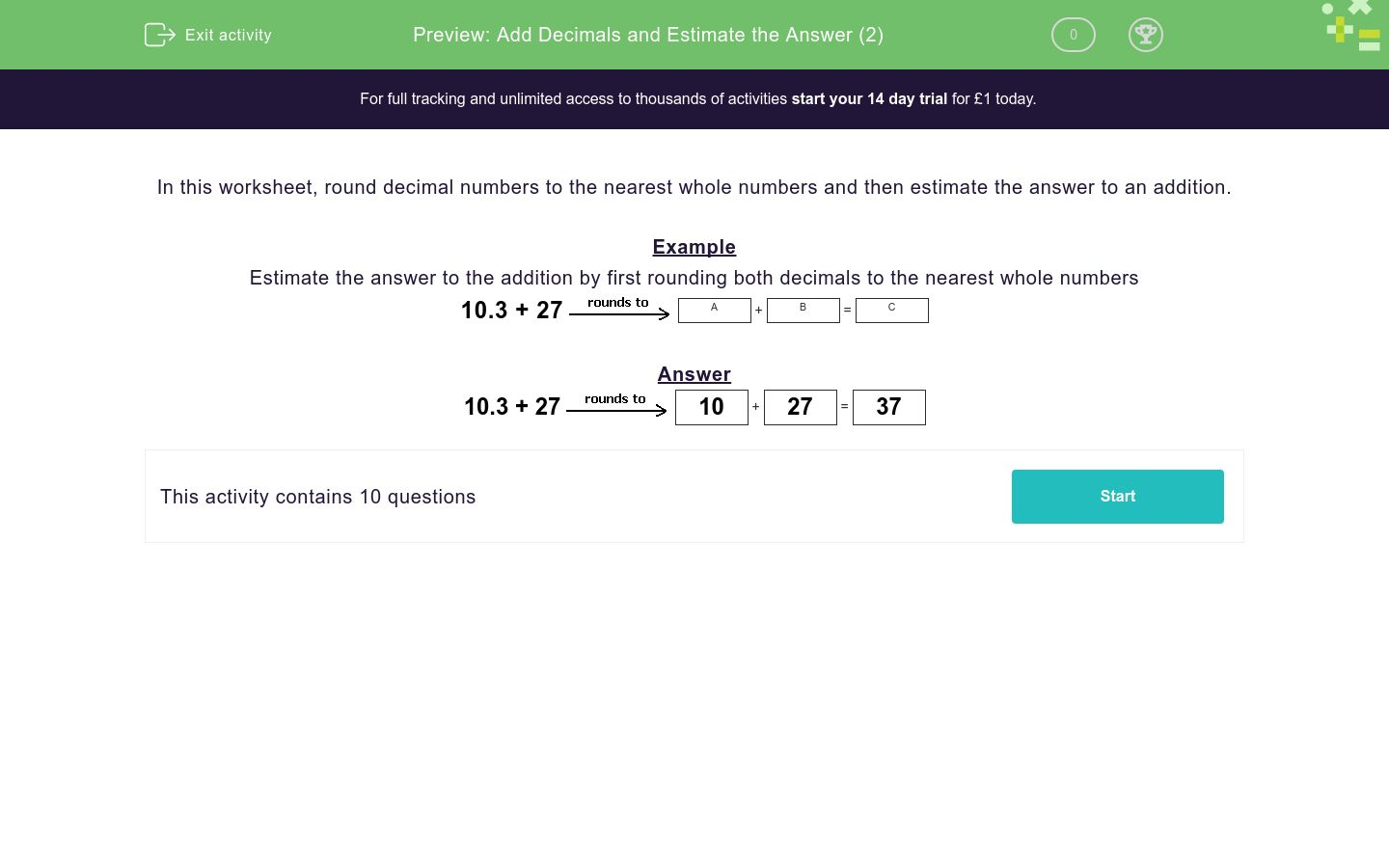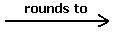### Affordable pricing from £10/month

In this worksheet, students round decimal numbers to the nearest whole numbers in order to estimate the answer to an addition.Key stage:  KS 2

Curriculum topic:   Number: Addition, Subtraction, Multiplication and Division

Curriculum subtopic:   Use Estimation to Check Answers

Difficulty level:### QUESTION 1 of 10

In this worksheet, round decimal numbers to the nearest whole numbers and then estimate the answer to an addition.

Example

Estimate the answer to the addition by first rounding both decimals to the nearest whole numbers

10.3 27A
+
 B
=
 C

10.3 2710
+
 27
=
 37

Estimate the answer by rounding the numbers to the nearest 100.

304 + 893A
+
 B
=
 C

Remember to put your answers in the same sequence as the question!

For example, A would be 304 to the nearest 100 and B would be 893...

## Column B

C
1200
A
900
B
300

Estimate the answer to the addition by first rounding both decimals to the nearest whole numbers.

27.8 + 15.4A
+
 B
=
 C

Remember to put your answers in the same sequence as the question!

For example, A would be 27.8 to the nearest whole number and B would be 15.4...

## Column B

A
28
B
15
C
43

Estimate the answer to the addition by first rounding both decimals to the nearest whole numbers.

11.8 + 10.5A
+
 B
=
 C

Remember to put your answers in the same sequence as the question!

For example, A would be 11.8 to the nearest whole number and B would be 10.5...

## Column B

A
12
B
23
C
11

Estimate the answer to the addition by first rounding both decimals to the nearest whole numbers.

68.6 + 58.7A
+
 B
=
 C

Remember to put your answers in the same sequence as the question!

## Column B

A
69
B
59
C
128

Estimate the answer to the addition by first rounding both decimals to the nearest whole numbers.

59.7 + 56.4A
+
 B
=
 C

Remember to put your answers in the same sequence as the question!

## Column B

A
56
B
60
C
116

Estimate the answer to the addition by first rounding both decimals to the nearest whole numbers.

57.6 + 47.8A
+
 B
=
 C

Remember to put your answers in the same sequence as the question!

## Column B

A
48
B
106
C
58

Estimate the answer to the addition by first rounding both decimals to the nearest whole numbers.

79.1 + 22.1A
+
 B
=
 C

Remember to put your answers in the same sequence as the question!

## Column B

A
22
B
79
C
101

Estimate the answer to the addition by first rounding both decimals to the nearest whole numbers.

26.7 + 22.6A
+
 B
=
 C

Remember to put your answers in the same sequence as the question!

## Column B

A
23
B
50
C
27

Estimate the answer to the addition by first rounding both decimals to the nearest whole numbers.

46.9 + 32.5A
+
 B
=
 C

Remember to put your answers in the same sequence as the question!

## Column B

A
33
B
47
C
80

Estimate the answer to the addition by first rounding both decimals to the nearest whole numbers.

94.9 + 31A
+
 B
=
 C

Remember to put your answers in the same sequence as the question!

## Column B

A
95
B
31
C
126
• Question 1

Estimate the answer by rounding the numbers to the nearest 100.

304 + 893A
+
 B
=
 C

Remember to put your answers in the same sequence as the question!

For example, A would be 304 to the nearest 100 and B would be 893...

## Column B

C
1200
A
300
B
900
EDDIE SAYS
300 + 900 = 1200
• Question 2

Estimate the answer to the addition by first rounding both decimals to the nearest whole numbers.

27.8 + 15.4A
+
 B
=
 C

Remember to put your answers in the same sequence as the question!

For example, A would be 27.8 to the nearest whole number and B would be 15.4...

## Column B

A
28
B
15
C
43
EDDIE SAYS
28 + 15 = 43
• Question 3

Estimate the answer to the addition by first rounding both decimals to the nearest whole numbers.

11.8 + 10.5A
+
 B
=
 C

Remember to put your answers in the same sequence as the question!

For example, A would be 11.8 to the nearest whole number and B would be 10.5...

## Column B

A
12
B
11
C
23
EDDIE SAYS
12 + 11 = 23
• Question 4

Estimate the answer to the addition by first rounding both decimals to the nearest whole numbers.

68.6 + 58.7A
+
 B
=
 C

Remember to put your answers in the same sequence as the question!

## Column B

A
69
B
59
C
128
EDDIE SAYS
69 + 59 = 128
• Question 5

Estimate the answer to the addition by first rounding both decimals to the nearest whole numbers.

59.7 + 56.4A
+
 B
=
 C

Remember to put your answers in the same sequence as the question!

## Column B

A
60
B
56
C
116
EDDIE SAYS
60 + 56 = 116
• Question 6

Estimate the answer to the addition by first rounding both decimals to the nearest whole numbers.

57.6 + 47.8A
+
 B
=
 C

Remember to put your answers in the same sequence as the question!

## Column B

A
58
B
48
C
106
EDDIE SAYS
58 + 48 = 106
• Question 7

Estimate the answer to the addition by first rounding both decimals to the nearest whole numbers.

79.1 + 22.1A
+
 B
=
 C

Remember to put your answers in the same sequence as the question!

## Column B

A
79
B
22
C
101
EDDIE SAYS
79 + 22 = 101
• Question 8

Estimate the answer to the addition by first rounding both decimals to the nearest whole numbers.

26.7 + 22.6A
+
 B
=
 C

Remember to put your answers in the same sequence as the question!

## Column B

A
27
B
23
C
50
EDDIE SAYS
27 + 23 = 50
• Question 9

Estimate the answer to the addition by first rounding both decimals to the nearest whole numbers.

46.9 + 32.5A
+
 B
=
 C

Remember to put your answers in the same sequence as the question!

## Column B

A
47
B
33
C
80
EDDIE SAYS
47 + 33 = 80
• Question 10

Estimate the answer to the addition by first rounding both decimals to the nearest whole numbers.

94.9 + 31A
+
 B
=
 C

Remember to put your answers in the same sequence as the question!

## Column B

A
95
B
31
C
126
EDDIE SAYS
95 + 31 = 126
---- OR ----

Sign up for a £1 trial so you can track and measure your child's progress on this activity.

### What is EdPlace?

We're your National Curriculum aligned online education content provider helping each child succeed in English, maths and science from year 1 to GCSE. With an EdPlace account you’ll be able to track and measure progress, helping each child achieve their best. We build confidence and attainment by personalising each child’s learning at a level that suits them.

Get started